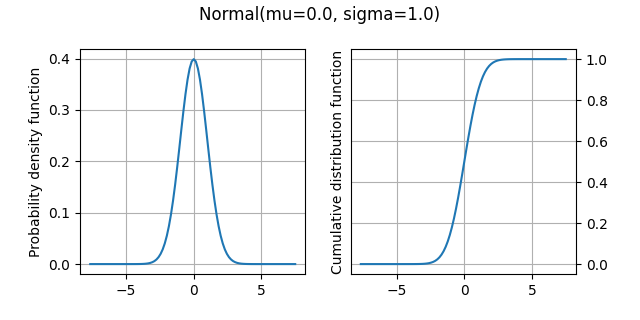# Probability distributions based on OpenTURNS¶

In this example, we seek to create a probability distribution based on the OpenTURNS library.

from __future__ import annotations

from gemseo import configure_logger
from gemseo.uncertainty import create_distribution
from gemseo.uncertainty import get_available_distributions

configure_logger()

<RootLogger root (INFO)>


First of all, we can access the names of the available probability distributions from the API:

all_distributions = get_available_distributions()
print(all_distributions)

['OTComposedDistribution', 'OTDiracDistribution', 'OTDistribution', 'OTExponentialDistribution', 'OTNormalDistribution', 'OTTriangularDistribution', 'OTUniformDistribution', 'SPComposedDistribution', 'SPDistribution', 'SPExponentialDistribution', 'SPNormalDistribution', 'SPTriangularDistribution', 'SPUniformDistribution']


and filter the ones based on the OpenTURNS library (their names start with the acronym ‘OT’):

ot_distributions = [dist for dist in all_distributions if dist.startswith("OT")]
print(ot_distributions)

['OTComposedDistribution', 'OTDiracDistribution', 'OTDistribution', 'OTExponentialDistribution', 'OTNormalDistribution', 'OTTriangularDistribution', 'OTUniformDistribution']


## Create a distribution¶

Then, we can create a probability distribution for a two-dimensional random variable whose components are independent and distributed as the standard normal distribution (mean = 0 and standard deviation = 1):

distribution_0_1 = create_distribution("x", "OTNormalDistribution", 2)
print(distribution_0_1)

Normal(mu=0.0, sigma=1.0)


or create another distribution with mean = 1 and standard deviation = 2 for the marginal distributions:

distribution_1_2 = create_distribution(
"x", "OTNormalDistribution", 2, mu=1.0, sigma=2.0
)
print(distribution_1_2)

Normal(mu=1.0, sigma=2.0)


We could also use the generic OTDistribution which allows access to all the OpenTURNS distributions but this requires to know the signature of the methods of this library:

distribution_1_2 = create_distribution(
"x", "OTDistribution", 2, interfaced_distribution="Normal", parameters=(1.0, 2.0)
)
print(distribution_1_2)

Normal(1.0, 2.0)


## Plot the distribution¶

We can plot both cumulative and probability density functions for the first marginal:

distribution_0_1.plot()<Figure size 640x320 with 2 Axes>


Note

We can provide a marginal index as first argument of the Distribution.plot() method but in the current version of GEMSEO, all components have the same distributions and so the plot will be the same.

## Get mean¶

We can access the mean of the distribution:

print(distribution_0_1.mean)

[0. 0.]


## Get standard deviation¶

We can access the standard deviation of the distribution:

print(distribution_0_1.standard_deviation)

[1. 1.]


## Get numerical range¶

We can access the range, ie. the difference between the numerical minimum and maximum, of the distribution:

print(distribution_0_1.range)

[array([-7.65062809,  7.65062809]), array([-7.65062809,  7.65062809])]


## Get mathematical support¶

We can access the range, ie. the difference between the minimum and maximum, of the distribution:

print(distribution_0_1.support)

[array([-inf,  inf]), array([-inf,  inf])]


## Generate samples¶

We can generate 10 samples of the distribution:

print(distribution_0_1.compute_samples(10))

[[ 0.60820165 -0.4705256 ]
[-1.2661731   0.26101794]
[-0.43826562 -2.29006198]
[ 1.2054782  -1.28288529]
[-2.18138523 -1.31178112]
[ 0.35004209 -0.09078383]
[-0.35500705  0.99579323]
[ 1.43724931 -0.13945282]
[ 0.81066798 -0.5602056 ]
[ 0.79315601  0.4454897 ]]


## Compute CDF¶

We can compute the cumulative density function component per component (here the probability that the first component is lower than 0. and that the second one is lower than 1.):

.. GENERATED FROM PYTHON SOURCE LINES 128-130

print(distribution_0_1.compute_cdf([0.0, 1.0]))

[0.5        0.84134475]


## Compute inverse CDF¶

We can compute the inverse cumulative density function component per component (here the quantile at 50% for the first component and the quantile at 97.5% for the second one):

print(distribution_0_1.compute_inverse_cdf([0.5, 0.975]))

[0.         1.95996398]


Total running time of the script: ( 0 minutes 0.185 seconds)

Gallery generated by Sphinx-Gallery